### Home > PC > Chapter 7 > Lesson 7.2.4 > Problem7-85

7-85.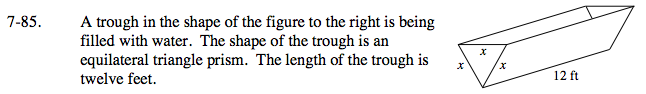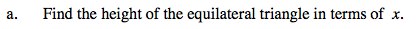Draw and label the triangle with information you know. Use the Pythagorean Theorem to solve for the height.

$\frac{\sqrt{3}}{2}x$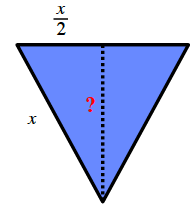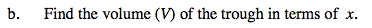V(x) = (area of the base)(length)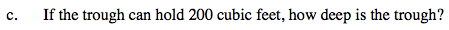Solve 200 = V(x).

h ≈ 5.373 ft